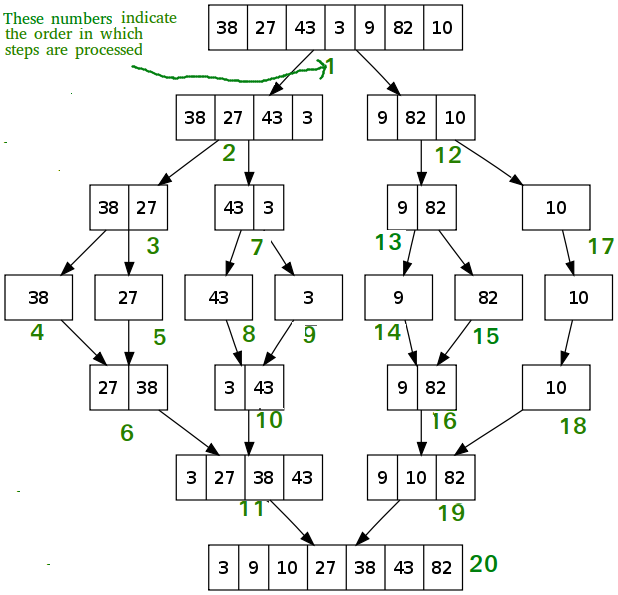# Merge Sort vs. Insertion Sort

Pre-requisite: Merge Sort, Insertion Sort

Merge Sort: is an external algorithm and based on divide and conquer strategy. In this sorting:

1. The elements are split into two sub-arrays (n/2) again and again until only one element is left.
2. Merge sort uses additional storage for sorting the auxiliary array.
3. Merge sort uses three arrays where two are used for storing each half, and the third external one is used to store the final sorted list by merging the other two and each array is then sorted recursively.
4. At last, all sub-arrays are merged to make it ‘n’ element size of the array.

Below is the image to illustrate Merge Sort:Insertion Sort is a sorting algorithm in which elements are taken from an unsorted item, inserting it in sorted order in front of the other items, and repeating until all items are in order. The algorithm is simple to implement and usually consists of two loops: an outer loop to pick items and an inner loop to iterate through the array. It works on the principle of the sorting playing cards in our hands.

Below is the image to illustrate Insertion Sort:Difference between Merge sort and Insertion sort:

• Time Complexity: In Merge Sort the Worst Case: O(N*log N), Average Case: O(N*log N), and Best Case: O(N*log N),
whereas
In Insertion Sort the Worst Case: O(N2), Average Case: O(N2), and Best Case: O(N).
• Space Complexity: Merge sort being recursive takes up the auxiliary space complexity of O(N) hence it cannot be preferred over the place where memory is a problem,
whereas
In Insertion sort only takes O(1) auxiliary space complexity. It sorts the entire array just by using an extra variable.
• Datasets: Merge Sort is preferred for huge data sets. It happens to compare all the elements present in the array hence is not much helpful for small datasets,
whereas
Insertion Sort is preferred for fewer elements. It becomes fast when data is already sorted or nearly sorted because it skips the sorted values.
• Efficiency: Considering average time complexity of both algorithm we can say that Merge Sort is efficient in terms of time and Insertion Sort is efficient in terms of space.
• Sorting Method: The merge sort is an external sorting method in which the data that is to be sorted cannot be accommodated in the memory and needed auxiliary memory for sorting,
whereas
Insertion sort is based on the idea that one element from the input elements is consumed in each iteration to find its correct position i.e., the position to which it belongs in a sorted array.
• Stability: Merge sort is stable as two elements with equal value appear in the same order in sorted output as they were in the input unsorted array,
whereas
Insertion sort takes O(N2) time on both data structures(Array and Linked list). If the CPU has an efficient memory block move function then the array may be quicker. Otherwise, there probably isn’t that much of a time difference.

Tabular Representation:

Parameters Merge Sort Insertion Sort
Worst Case Complexity O(N*log N) O(N2)
Average Case Complexity O(N*log N) O(N2)
Best Case Complexity O(N*log N) O(N)
Auxiliary Space Complexity O(N) O(1)
Works well on On huge dataset. On small dataset.
Efficiency Comparitively Efficient. Comparitively Inefficient.
Inplace Sorting No Yes
Algorithm Paradigm Divide and Conquer Incremental Approach
Uses It is used for sorting linked list in O(N*log N), for Inversion Count problem, External sorting, etc. It is used when number of elements is small. It can also be useful when input array is almost sorted, only few elements are misplaced in complete big array.My Personal Notes arrow_drop_upCheck out this Author's contributed articles.

If you like GeeksforGeeks and would like to contribute, you can also write an article using contribute.geeksforgeeks.org or mail your article to contribute@geeksforgeeks.org. See your article appearing on the GeeksforGeeks main page and help other Geeks.

Please Improve this article if you find anything incorrect by clicking on the "Improve Article" button below.

1

Please write to us at contribute@geeksforgeeks.org to report any issue with the above content.